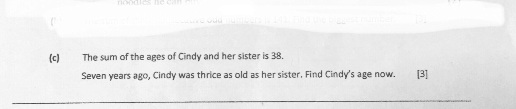# QuestionAnyone help me with attached question

Thanks

1 Answer

# Answer

This is a Simultaneous Equation question.

Let Cindy be x years, sister be y years old.

x + y = 38

x = 38 – y  (1)

7 year ago,     (amendments )

(x – 7) / 3 = (y – 7) / 1

x – 7 = 3y – 21  (2)

Subt (1) into (2)

38 – y – 7 = 3y – 21

31 + 21 = 3y + y

4y = 52

y = 52 ÷ 4 = 13

x = 38 – 13 = 25 year old

1 Reply 1 Like ✔Accepted Answer

Thanks for solution. But answer was given 25 years old.

please advise.

1 Reply 0 Likes